Function Repository Resource:

# Fusc

Evaluate the fusc function

Contributed by: Christopher Stover
 ResourceFunction["Fusc"][n] gives the fusc function fusc(n).

## Details

Integer mathematical function, suitable for both symbolic and numerical manipulation.
ResourceFunction["Fusc"] is defined by the recurrence relation fusc(0)=0, fusc(1)=1, fusc(2m)=fusc(m) and fusc(2m+1)=fusc(m)+fusc(m+1).
According to author Edsger W. Dijkstra (EWD 570), the fusc function is named for the obfuscated appearance of its output.

## Examples

### Basic Examples (3)

Evaluate numerically for positive even integer input:

 In:=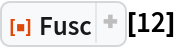Out=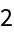Evaluate numerically for positive odd integer input:

 In:=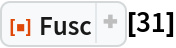Out=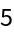Plot Fusc over a subset of the non-negative integers:

 In:=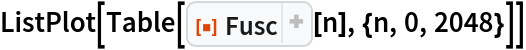Out=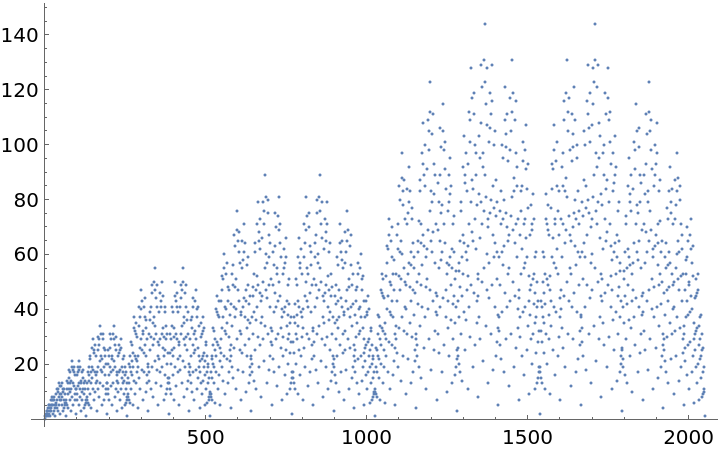### Scope (1)

 In:=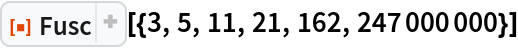Out=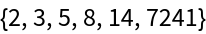### Applications (4)

The value fusc(n+1) is the number of odd binomial coefficients of the form Binomial[n-r,r], 02rn:

 In:=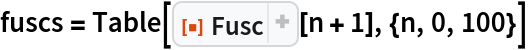Out=In:=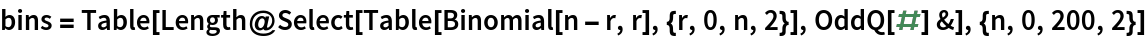Out=Confirm the two lists are equal:

 In:=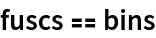Out=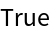An alternative way to compute the same quantity:

 In:=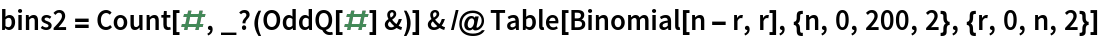Out=Confirm all three are equal:

 In:=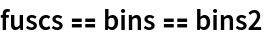Out=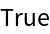### Possible Issues (1)

Fusc returns unevaluated if its input is anything other than a non-negative integer:

 In:=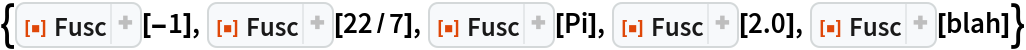Out=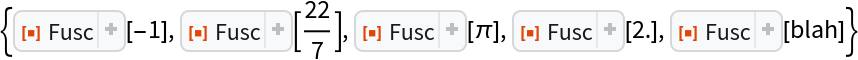### Neat Examples (3)

Generate the first 100 terms of Stern's diatomic series, also known as the Stern–Brocot sequence (OEIS A002487):

 In:=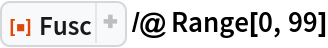Out=When used in conjunction with Prime, you can generate OEIS sequences A261179 and A261273:

 In:=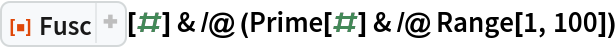Out=In:=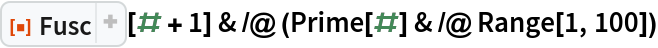Out=These two sequences combine to form the prime numbered elements in the Calkin–Wilf sequence, one popular enumeration of the rational numbers. More precisely, the terms of the Calkin–Wilf enumeration of the rationals are given by fusc(n)/fusc(n+1):

 In:=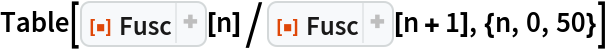Out=therealcstover

## Version History

• 1.0.0 – 09 September 2022